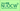# Calculus ILecturer Futaba FUJIE, Associate Professor Institute of Liberal Arts & Sciences, 2015 Spring School of Science (2・週1回全15回)

### Key Features

Although basics of single variable calculus are covered in high school mathematics, there are actually a lot more to consider in order to really understand the subject. For example, what does it really mean that a function is continuous at a certain point? While graphs are helpful, there are situations where the function in question is so complicated that it is impossible to draw its graph. Intuition is important, but it is essential to understand how things are mathematically defined and use them as building blocks. Learning mathematics is in some sense learning foreign languages. By going through proofs of theorems together in classroom, I hope that you feel comfortable with "math grammar" so that you can enjoy mathematics more.

### Course Objectives

Analysis is the field of mathematics that describes and analyzes quantitative changes and its central method is differential and integral calculus. While calculus is an essential research tool in natural sciences, it has recently found a number of applications in social sciences as well. The aim of the first half of this one-year course is to provide a solid understanding of single variable calculus.

### Course Content

The topics covered in this course are listed below.

1. - the limit of a sequence,the limit of a function and its continuity
2. key words:

• the limit of a sequence,

• the limit of a function,

• the monotone convergence theorem,

• basic properties of continuous functions

3. differential calculus of one variable
4. key words:

• the derivative of a function and its geometric properties,

• basic properties of derivatives of functions,

• the inverses and derivatives of elementary functions,

• the mean value theorem,

• derivatives of higher order,

• Taylor's theorem,

• the limit of an indeterminate form

5. integral calculus of one variable
6. key words:

• definite integral,

in- definite integral,

• the fundamental theorem of calculus,

• improper integral

#### Last updated

May 16, 2020

OCW website, and all course materials made public on this site,
may be used under the terms and conditions posted under "About OCW"
© 2020 Nagoya University Open Course Ware